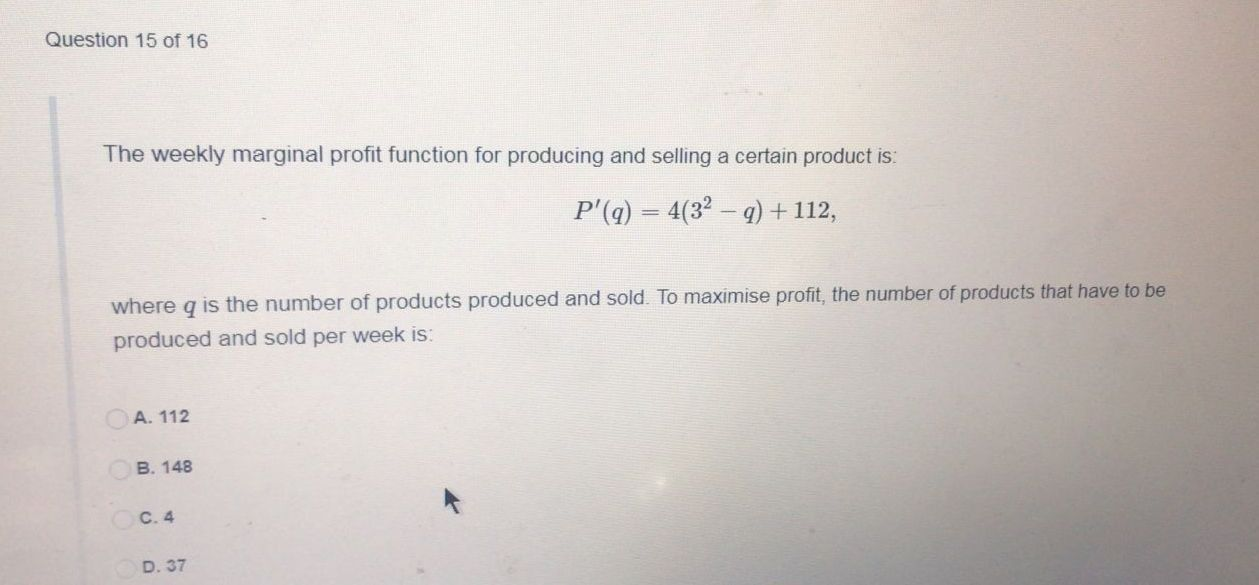### Still have math questions?

Algebra
QuestionThe weekly marginal profit function for producing and selling a certain product is:

$$P ^ { \prime } ( q ) = 4 ( 3 ^ { 2 } - q ) + 112$$

where $$q$$ is the number of products produced and sold. To maximise profit, the number of products that have to be produced and sold per week is:

A. $$112$$

B. $$148$$

C. $$4$$

D. $$37$$